Tamilnadu State Board New Syllabus Samacheer Kalvi 12th Maths Guide Pdf Chapter 11 Probability Distributions Ex 11.3 Textbook Questions and Answers, Notes.

## Tamilnadu Samacheer Kalvi 12th Maths Solutions Chapter 11 Probability Distributions Ex 11.3

Question 1.
The probability density function of X is given by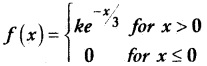Find the value of k.
Solution:
Since f(x) is a probability density function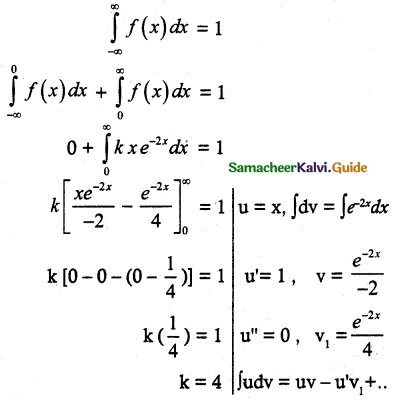Question 2.
The probability density function of X is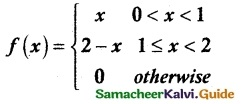Find
(i) P(0.2 ≤ X < 0.6)
(ii) P(1.2 ≤ X < 1.8)
(iii) P(0.5 ≤ X < 1.5)
Solution: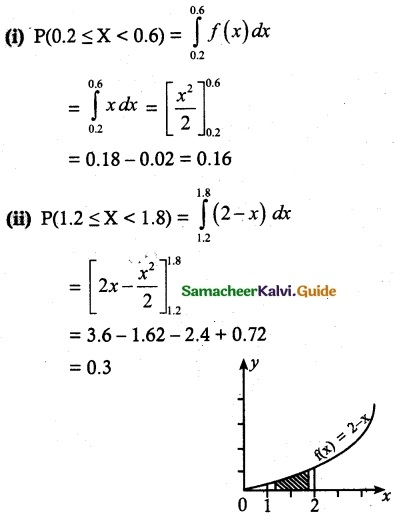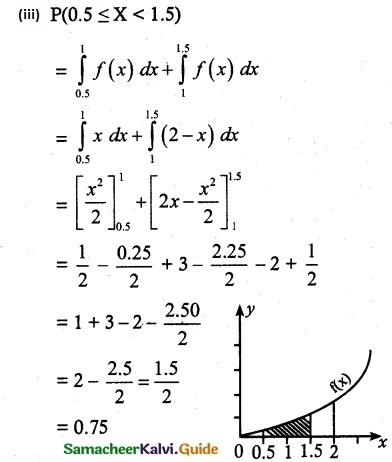Question 3.
Suppose the amount of milk sold daily at a milk booth is distributed with a minimum of 200 litres and a maximum of 600 litres with probability density function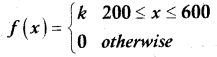Find
(i) the value of k
(ii) the distribution function
(iii) the probability that daily sales will fall between 300 litres and 500 litres?
Solution: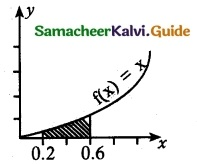(i) Since f(x) is a probability density function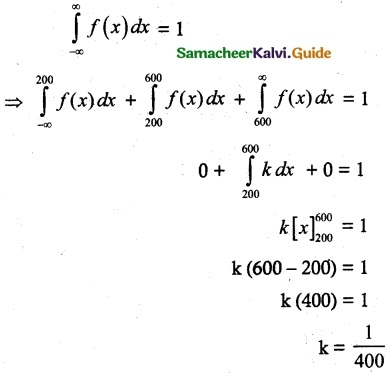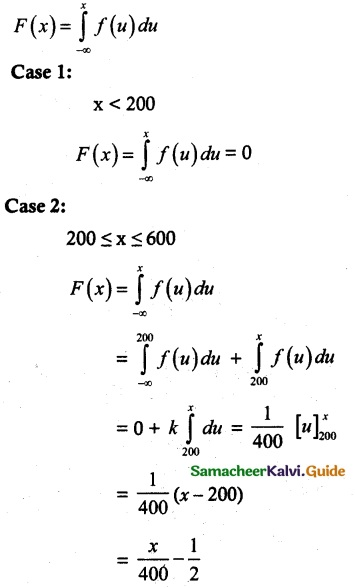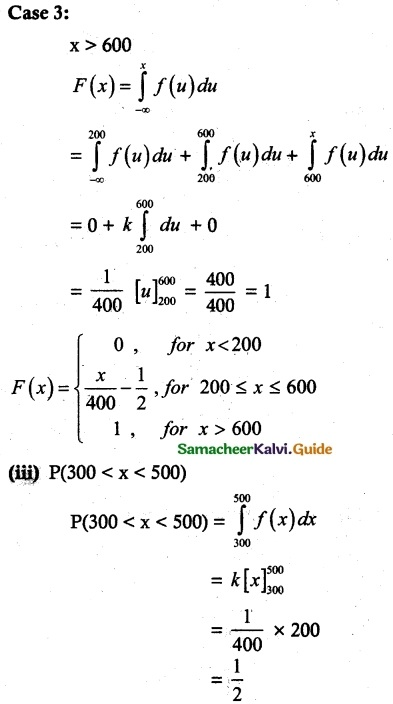Question 4.
The probability density function of X is given by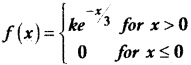Find
(i) the value of k
(ii) the distribution function
(iii) P(X < 3)
(iv) P(5 ≤ X)
(v) P(X ≤ 4)
Solution:
(i) Since f is a probability density function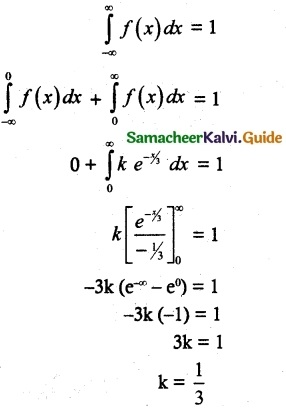(ii) The distribution function F(x) = $$\int_{-\infty}^{x}$$ f(u) du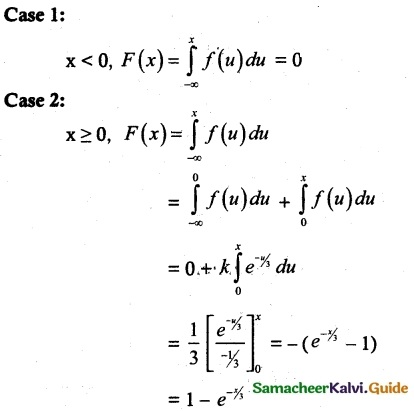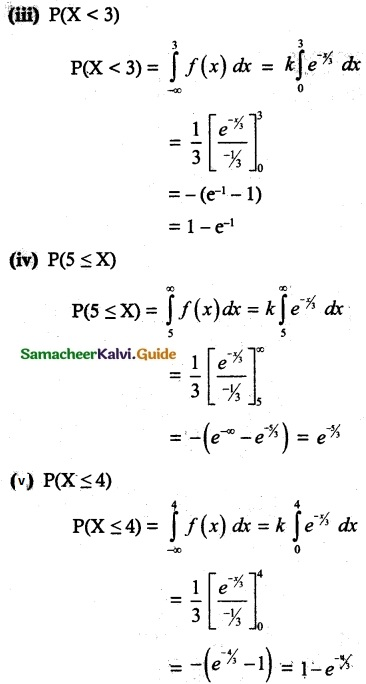Question 5.
If X is the random variable with probability density function f(x) given by,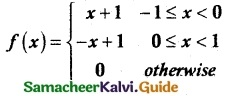then find
(i) the distribution function F(x)
(ii) P(-0.5 ≤ x ≤ 0.5)
Solution:
Distribution function F(x) = $$\int_{-\infty}^{x}$$ f(u) du
case 1.
x < -1
F(x) = $$\int_{-\infty}^{x}$$ f(u) du = 0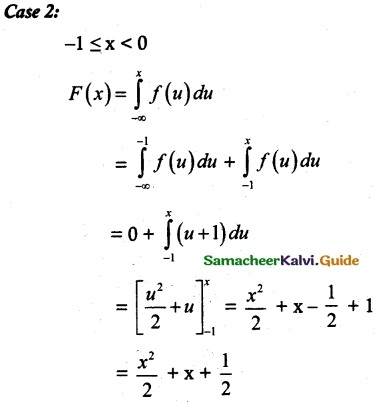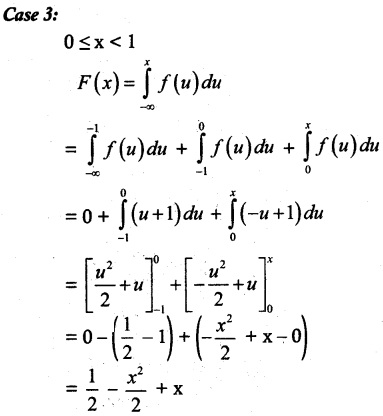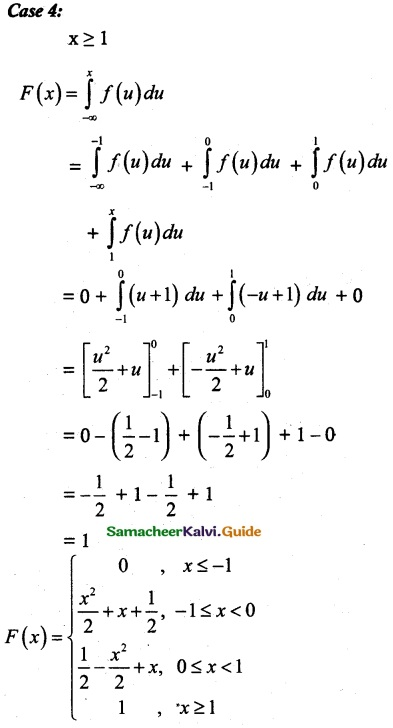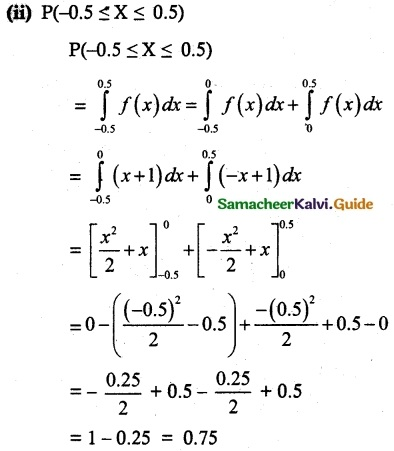Question 6.
If X is the random variable with distribution function F(x) given by,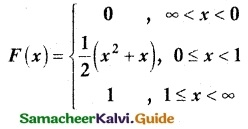Find
(i) the probability density function f(x)
(ii) P(0.3 ≤ X ≤ 0.6)
Solution:
(i) Probability density function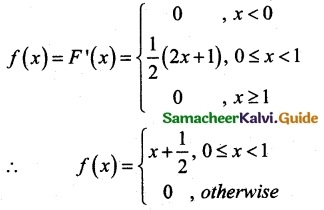(ii) P(0.3 ≤ X ≤ 0.6)
P(a ≤ X ≤ b) = F(b) – F(a)
P(0.3 ≤ X ≤ 0.6) = F(0.6) – F(0.3)
= $$\frac { 1 }{ 2 }$$ [(0.6)² + (0.6)] – $$\frac { 1 }{ 2 }$$ [(0.3)² + (0.3)]
= $$\frac { 1 }{ 2 }$$ (0.96 – 0.39)
= 0.285